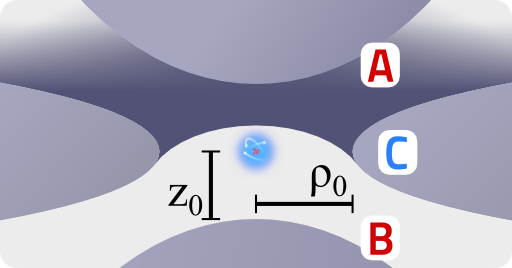Taming Ions: the Paul TrapIn this problem we want to understand the mechanism behind ion trapping. We will study the movement of charged particles in a so-called Paul trap by solving the equations of motion using approximative methods.

Problem StatementWolfgang Paul received 1989 the Nobel prize in physics for the invention of the so-called Paul trap. Such a device is generally made of three elements of hyperboloidal shape: a ring around the $$z$$ axis of symmetry with innermost radius $$\rho_{0}$$ and two electrodes starting at $$\pm z_{0}$$, see figure on the right. The two electrodes, A and B, are on a different potential than the ring C. Such a configuration yields a quadrupolar electrostatic potential inside the Paul trap: $\phi\left(\mathbf{r};t\right) = \phi_{0}\left(t\right)\frac{\rho^{2}-2z^{2}}{\rho_{0}^{2}+2z_{0}^{2}}\ .$As we can see, there is an explicit time-dependent function involved, for which we want to assume a slow oscillation with a constant (dc) shift, i.e. $\phi_{0}\left(t\right) = U_{\mathrm{dc}}-U_{\mathrm{ac}}\,\cos\left(\Omega t\right)\ .$In the following we will convince ourselves that such a potential can indeed be used for ion trapping:

1. Show that the equations of motion for a charged particle with charge $$q$$ and mass $$m$$ can be cast in the normal form of a Mathieu differential equation,$\frac{d^{2}u\left(\tau\right)}{d\tau^{2}}+\left[a_{u}-2q_{u}\cos\left(2\tau\right)\right]u\left(\tau\right) = 0\ ,$ for which $$u\in\left\{ \rho,z\right\}$$ and identify the dimensionless parameters $$a_{u}$$, $$q_{u}$$ and $$\tau$$.
2. Find an approximate solution to the Mathieu differential equation for $$U_\mathrm{dc}=0$$. Express the motion of the particle as a superposition of a fast background and slow overall motion of it using $u\left(\tau\right) = u_{0}\left(\tau\right)+\delta u\left(\tau\right)$with the assumptions$\begin{eqnarray*} \left|u_{0}\left(\tau\right)\right|&\gg&\left|\delta u\left(\tau\right)\right|\ \mathrm{and}\\\left|\frac{d^{n}u_{0}\left(\tau\right)}{d\tau^{n}}\right|&\ll&\left|\frac{d^{n}\delta u\left(\tau\right)}{d\tau^{n}}\right|\ \forall n\geq1\ . \end{eqnarray*}$

Background: The numerous Applications of the Paul Trap

We have just calculated that the trapping mechanism really works and hopefully got a basic feeling for the situation. But there is, of course much more to talk about. First of all, if we consider the possible applications of ion traps, the study of fundamental interactions of particles comes to mind. This can be done, since the interaction between different charged particles or to external fields compared to the interaction to the trap itself is generally much stronger. This is employed e.g. in atomic clocks where the trap is an important part that must not change the internal energetic structure of the ions. Below you can find a very nice explanation of atomic clocks by the 2012 Nobel prize winner David Wineland. He goes into detail on trapping after about thirty minutes, if you want to fast forward:

Another possibility to use ion traps is to employ them as a mass spectrometer. We haven't talked about this specific issue so far, because this application relies on a certain property of the solutions of the Mathieu equation: Roughly speaking, if the parameters $$a_{u}$$ and $$q_{u}$$ have certain values, trapping is possible, otherwise the motion is unstable. Now since these parameters directly depend on the mass of the ions and the parameter regions of stability are well-known, the Paul trap can be used as a mass spectrometer! There is a very nice review by Raymond March in the Journal of Mass Spectrometry 32 (1997) “An Introduction to Quadrupole Ion Trap Mass Spectrometry”.

A very convenient introduction to the physics of trapping is also provided in the Nobel lecture by Wolfgang Paul himself entitled “Electromagnetic traps for charged and neutral particles” (1989).